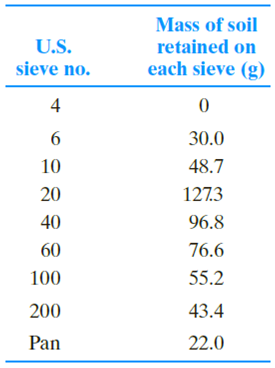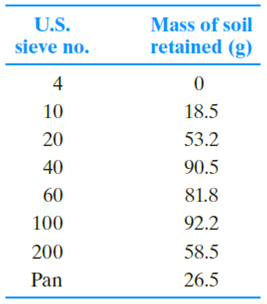Chapter 2, Problem 2.5PPrinciples of Geotechnical Enginee...

9th Edition
Braja M. Das + 1 other
ISBN: 9781305970939

Solutions

Chapter
SectionPrinciples of Geotechnical Enginee...

9th Edition
Braja M. Das + 1 other
ISBN: 9781305970939
Textbook Problem

Repeat Problem 2.4 with the following data.2.4 The following are the results of a sieve analysis.a. Determine the percent finer than each sieve and plot a grain-size distribution curve.b. Determine D10, D30, and D60 for each soil.c. Calculate the uniformity coefficient Cu.d. Calculate the coefficient of gradation Cc.

(a)

To determine

Calculate the percent finer than each sieve and plot a grain-size distribution curve.

Explanation

Calculation:

Calculate the total mass of the soil (M) using the relation.

M=M1+M2++Mn+Mp

Here, M1+M2++Mn is mass of soil retain in corresponding sieve number and Mp is the mass of soil retained in the pan.

Refer the given table for mass of soil retained in each sieve no.

Calculate the total mass of the soil as follows:

M=0+30+48.7+127.3+96.8+76.6+55.2+43.4+22=500g

Calculate the cumulative mass retained on each sieve using the relation.

Mi=M1+M2++Mn

For mass 1 (M1):

M1=M1=0

For mass 2 (M2):

M2=M1+M2=0+30=30g

For mass 3 (M3):

M3=M1+M2+M3=0+30+48

(b)

To determine

Calculate D10,D30, and D60 for each soil.

(c)

To determine

Calculate the uniformity coefficient.

(d)

To determine

Calculate the coefficient of gradation.

Still sussing out bartleby?

Check out a sample textbook solution.

See a sample solution

The Solution to Your Study Problems

Bartleby provides explanations to thousands of textbook problems written by our experts, many with advanced degrees!

Get Started

Why is important to know integral calculus?

Engineering Fundamentals: An Introduction to Engineering (MindTap Course List)

Give an example of each of the three types of relationships.

Database Systems: Design, Implementation, & Management

What do the two pressure gauges on a regulator show?

Welding: Principles and Applications (MindTap Course List)

Explain the use of permissions on a network.

Enhanced Discovering Computers 2017 (Shelly Cashman Series) (MindTap Course List)

What is a twist ratio, and why is it important?

Network+ Guide to Networks (MindTap Course List)